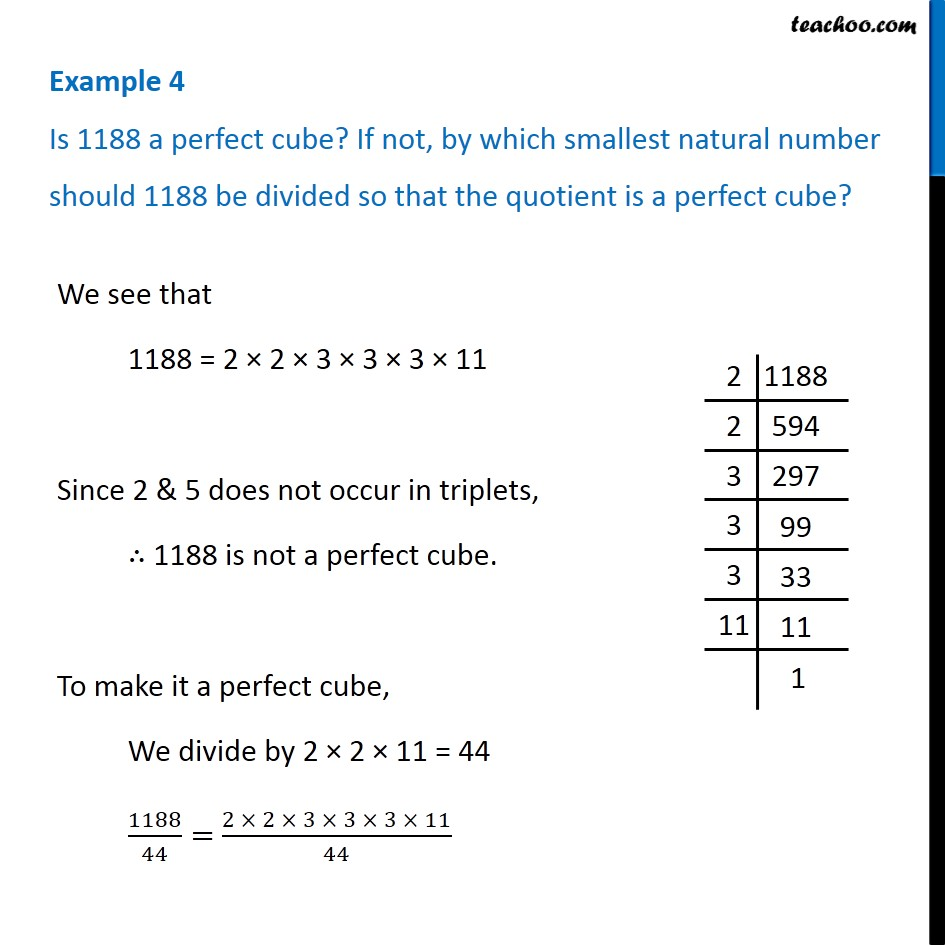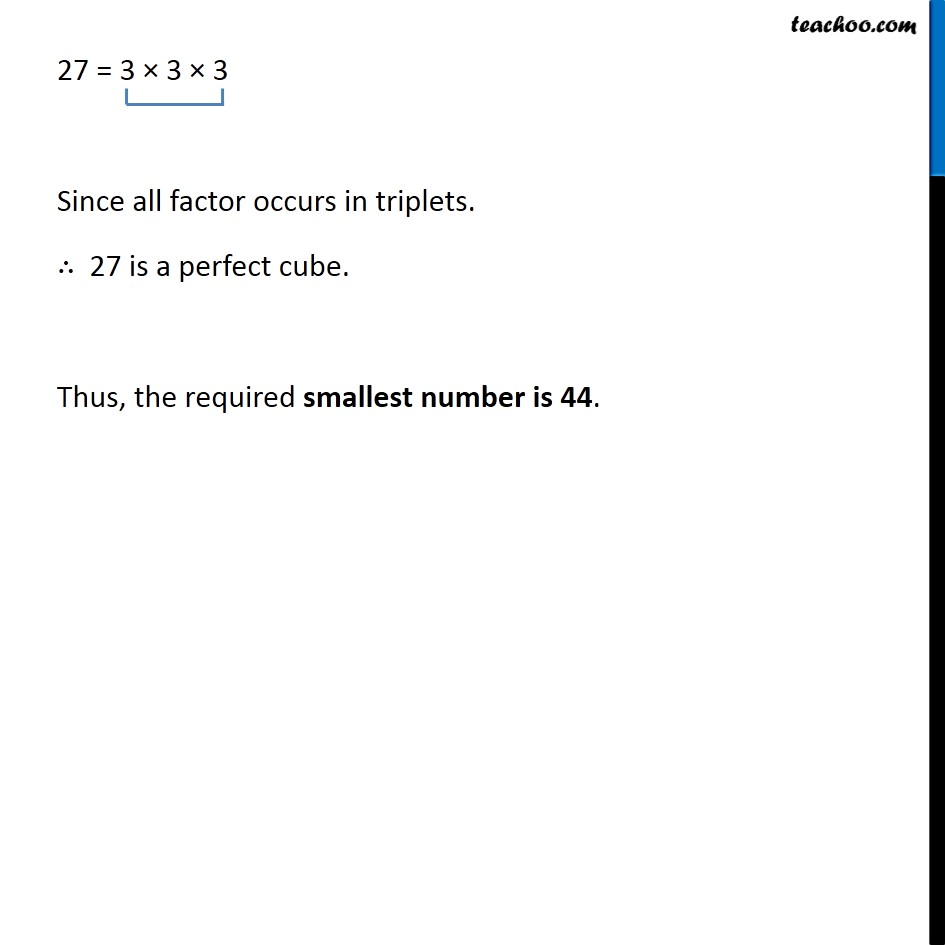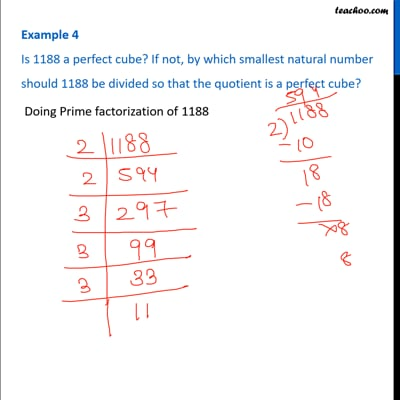Examples

Chapter 7 Class 8 Cubes and Cube Roots
Serial order wiseThis video is only available for Teachoo black users

### Transcript

Example 4 Is 1188 a perfect cube? If not, by which smallest natural number should 1188 be divided so that the quotient is a perfect cube? We see that 1188 = 2 × 2 × 3 × 3 × 3 × 11 Since 2 & 5 does not occur in triplets, ∴ 1188 is not a perfect cube. To make it a perfect cube, We divide by 2 × 2 × 11 = 44 1188/44= (2 × 2 × 3 × 3 × 3 × 11)/44 27 = 3 × 3 × 3 Since all factor occurs in triplets. ∴ 27 is a perfect cube. Thus, the required smallest number is 44.